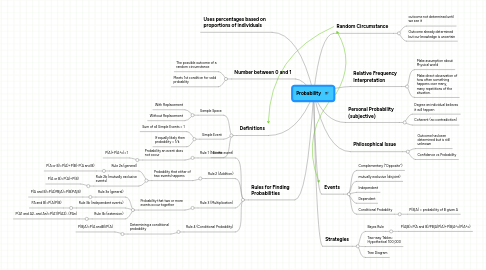Probability

Get Started. It's FreeProbability6. Definitions

6.1. Sample Space

6.1.1. With Replacement

6.1.2. Without Replacement

6.2. Simple Event

6.2.1. Sum of all Simple Events = 1

6.2.2. If equally likely then probability = 1/k

7. Events

7.5. Conditional Probablity

7.5.1. P(B|A) = probability of B given A

8. Rules for Finding Probabilities

8.1. Rule 1 (Not the event)

8.1.1. Probability an event does not occur

8.1.1.1. P(A)+P(A^c)=1

8.2. Rule 2 (Addition)

8.2.1. Probability that either of two events happens

8.2.1.1. Rule 2a (general)

8.2.1.1.1. P(A or B)=P(A)+P(B)-P(A and B)

8.2.1.2. Rule 2b (mutually exclusive events)

8.2.1.2.1. P(A or B)=P(A)+P(B)

8.3. Rule 3 (Multiplication)

8.3.1. Probability that two or more events occur together

8.3.1.1. Rule 3a (general)

8.3.1.1.1. P(A and B)=P(A)PB|A)=P(B)PA|B)

8.3.1.2. Rule 3b (independent events)

8.3.1.2.1. PA and B)=P(A)P(B)

8.3.1.3. Rule 3b (extension)

8.3.1.3.1. P(A1 and A2...and An)=P(A1)P(A2)...(PAn)

8.4. Rule 4 (Conditional Probability)

8.4.1. Determining a conditional probability

8.4.1.1. P(B|A)=P(A andB)/P(A)

10. Strategies

10.1. Bayes Rule

10.1.1. P(A|B)=P(A and B)/PB|A)P(A)+P(B|A^c)P(A^c)